## D-C Meter Formulas

Voltage Multipliers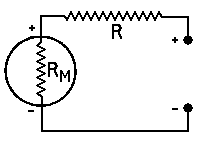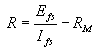R = multiplier resistance in ohms,
Efs = full scale reading required in volts,
Ifs = full scale current of meter in am-
peres,
RM = meter resistance in ohms
Measuring Resistance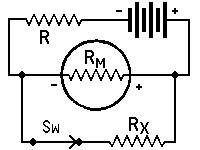with Milliammeter and battery*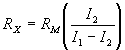RX = unknown resistance in ohms,
RM = meter resistance in ohms, or effec-
tive meter resistance if a shunted
range is used,
I1 = current reading with switch open,
I2 = current reading with switch closed,
RL = current limiting resistor of suffi-
cient value to keep meter reading
on scale when switch is open
*Approximately true only when current limiting
resistor is large as compared to meter resistance.

 FULL SCALECURRENT SHUNTRESISTANCE 0-10   ma 0-50   ma 0-100 ma 0-500 ma 3.0       ohms 0.551   ohms 0.272   ohms 0.0541 ohms

Measuring Resistance--(Continued)with Milliammeter, Battery and Known ResistorRX = unkown resistance in ohms,
RY = kown resistance in ohms,
RM = meter resistance in ohms,
I1 = current reading with switch closed,
I2 = current reading with switch open,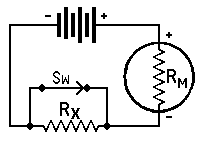with voltmeter and BatteryRX = unkown resistance in ohms,
RM = meter resistance in ohms, including
multiplier resistance if a multiplied
range is used,
E1 = voltmeter reading with switch closed,
E2 = voltmeter reading with switch open,

 FULL SCALEVOLTAGE MULTIPLIERRESISTANCE 0-10      volts 0-50      volts 0-100    volts 0-250    volts 0-500    volts 0-1,000 volts 10,000 ohms       50,000 ohms     100,000 ohms     250,000 ohms     500,000 ohms  1,000,000 ohms